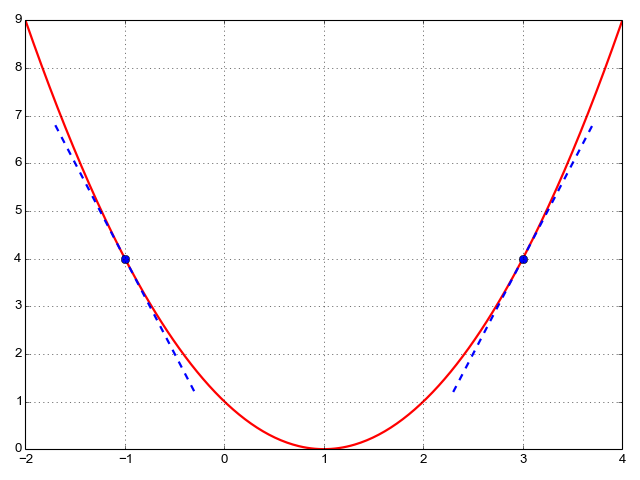# Lesson 4 apply_step function: Why do we always subtract the gradients from the params?

Here is the definition from chapter 4:

``````def apply_step(params, prn=True):
preds = f(time, params)
loss = mse(preds, speed)
loss.backward()
params.data -= lr * params.grad.data <---------------
if prn: print(loss.item())
return preds
``````

My question is: why do we always subtract the gradients from the params? Sometimes the gradients are all positive, sometimes they are negative. Wouldn’t subtracting always result in one stepping the wrong direction?

Thank you.

Hello,

In the graph below (source), the red curve is akin to the loss function, the X-axis denotes a single parameter, and the blue tangents are the gradients.Let’s consider x = -1: It is clear that increasing x (up to x = 1) would reduce the loss, but by adding the slope of the corresponding tangent to it, which is negative, x would actually become smaller, resulting in a greater loss. On the other hand, subtracting the slope from x would push it nearer x = 1 because the negative of a negative is positive.

Conversely, for x = 3, the slope of the tangent line is positive, and therefore x would move further away from the minimum if the two are summed. Instead, the slope is subtracted from x, thereby pulling it towards x = 1 and thus minimizing the loss.

2 Likes

Super clear explanation + visual. That makes a ton of sense. This might be a good explanation to have in the book…

Thank you Borna!!

2 Likes